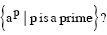# GATE | GATE CS 2008 | Question 8

Which of the following is true for the language(A) It is not accepted by a Turing Machine
(B) It is regular but not context-free
(C) It is context-free but not regular
(D) It is neither regular nor context-free, but accepted by a Turing machine

Explanation: Turing machine can be designed for ap using the concept of ‘Sieve of Eratosthenes’.

Suppose we are given an integer ‘n’ and we want to find out all the prime numbers less than or equal to ‘n’.

We repeat the following steps :
We find the smallest number in the list, declare it prime and eliminate all the multiples of that number from the list. We keep doing this until each element has been declared prime or eliminated from the list.

Now, if p = 0 or p = 1, we reject the input.
Else, we replace the first and the last ‘a’ with symbol \$.

In the above steps, what we do is we find the first non-black symbol from the left. Let this symbol occur at position ‘x’. Suppose ‘x’ is a prime number.
If this non-blank symbol is \$, input string will be accepted.
But, if the symbol is ‘a’, we mark it as a* and replace all the multiples of ‘x’ with the symbol ‘blank’.
If at the end, symbol \$ is replaced with ‘blank’, we reject the input string (because p will be multiple of some ‘x’ in that case).
Else, we go back and repeat the steps.

Thus, the input is neither regular nor context-free, but is accepted by a Turing machine.

Please comment below if you find anything wrong in the above post.

Quiz of this Question

My Personal Notes arrow_drop_up
Article Tags :

Be the First to upvote.

Please write to us at contribute@geeksforgeeks.org to report any issue with the above content.